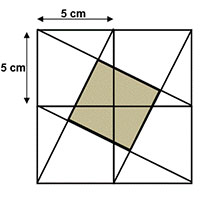Welcome to week 4 of our weekly maths challenge, with problems and puzzles posed by Gordon Burgin, Andrew Holt and the U3A Maths and Stats Subject Adviser - David Martin.

If you would like to share your ideas on how to solve these puzzles please join our learning forum or discuss within your U3A and interest group. The solutions to week two’s problems are below. Check back each week for the solutions - Week 3 solutions are at the bottom of the page - and let us know how you get on by contacting This email address is being protected from spambots. You need JavaScript enabled to view it.. New maths puzzles will go up onto the website every Thursday.

### Week Four

Question 1

In the addition sum below, A, B, C & D represent different digits, and all the digits in the sum are different. What is the sum of A, B, C & D?

5A
BC
D43

Question 2

You cash your pay cheque and save half of it. With the rest you pay a debt of £20 and buy something for £5. After spending 1/5 of the remaining money you are left with £12. How much was your pay?

Question 3

What is the area of the shaded square formed within a 10cm square?Question 4

I have a wooden right circular cone with height H and radius of base R. What is the greatest volume right circular cylinder I can cut out of this?

### Solutions to Week 3

Week Three

Question 1
In a batch of 8 correctly manufactured balls , a faulty, slightly lighter ball has
weights how could the lighter ball be identified and what is the minimum
number of weighings needed?

Solution
First consider the simpler case of just 3 balls of which one is known to be
lighter. Place any 2 of these balls on the scales. Either this identifies the lighter
ball or they balance in which case the third ball is the lighter one. For nine
balls, first divide them into three sets of 3. Then proceed as above to establish
which set of three contains the lighter ball; and then repeat with this set of
balls to find the lighter ball. We need then just 2 weighings to identify the lighter ball.

Question 2
In ‘Die Hard with a Vengeance’ Bruce Willis and Samuel L. Jackson were called
upon to measure out exactly 4 gallons of water at a fountain to defuse a bomb.
They were to use just a 3 gallon container and a 5 gallon container. How would
you have solved this puzzle? Can you find a way of measuring out all amounts
from 1 gallon to 8 gallons?
Solution
The answers are set out in terms of the contents of the 5 and 3 gallon
containers (in that order) at each stage of the operation with the final position in bold.
To get to 4:
(5, 0) (2, 3) (2, 0) (0,2) (5,2) (4, 3)
For other numbers:
1: (0 ,3) (3 , 0) (3, 3) (5, 1)
2: (5 ,0) (2, 3)
3: (0 , 3)
5: (5 , 0)
6: (0 , 3) (3 , 0) (3 , 3)
7. (5 ,0) (2, 3) (2 , 0) (0 ,2) (5, 2)
8. (5,3)

Question 3
Show that if p is a prime greater than 3 then p2 – 1 is divisible by 24

Solution
Primes greater than 3 have the form 6n ± 1. This is because 6n ± 2 is even and 6n + 3 is divisible by 3.
If p = 6n ± 1 then p2 – 1 = 36n2 ± 12n + 1 – 1
= 36n2 ± 12n
= 12n (3n ±1)
If n is even then 12n is divisible by 24.
If n is odd then 3n ± 1 is even and so 12(3n ± 1) is divisible by 24.

Question 4
What is the probability of getting a one pair hand in poker?

Solution

There are 52 C 5 = 52 x 51 x 50 x 49 x 48 possible hands
There are 13 values a card can take and 4C2 = 6 ways a pair can be formed from the 4 suits. So the number of pairs is 13 x 6
The remaining cards have to be distinct by value and there are 12C3 ways this can happen and each of these can come from the 4 different suits. So there are 12C3 x 43 ways this can occur.
So the probability of a one pair hand is (78 x 12C3 x 43)/52C5 = 1098420/2598960 ≈ 0.42268
This frequency is higher than one might intuitively expect.

How did you do?  Let us know at This email address is being protected from spambots. You need JavaScript enabled to view it.